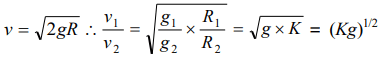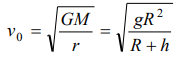## Gravitation Questions and Answers Part-18

1. For a satellite moving in an orbit around the earth, the ratio of kinetic energy to potential energy is
a) 2
b) $\frac{1}{2}$
c) $\frac{1}{\sqrt{2}}$
d) $\sqrt{2}$

Explanation:2.The value of escape velocity on a certain planet is 2 km/s. Then the value of orbital speed for a satellite orbiting close to its surface is
a) 12 km/s
b) 1 km/s
c) $\sqrt{2} km/s$
d) $2\sqrt{2} km/s$

Explanation:3. Four particles each of mass M, are located at the vertices of a square with side L. The gravitational potential due to this at the centre of the square is
a) $-\sqrt{32}\frac{GM}{L}$
b) $-\sqrt{64}\frac{GM}{L^{2}}$
c) Zero
d) $\sqrt{32}\frac{GM}{L}$

Explanation:4. There are two planets. The ratio of radius of the two planets is K but ratio of acceleration due to gravity of both planets is g. What will be the ratio of their escape velocity
a) $\left(kg\right)^{1/2}$
b) $\left(kg\right)^{-1/2}$
c) $\left(kg\right)^{2}$
d) $\left(kg\right)^{-2}$

Explanation:5. Which one of the following statements regarding artificial satellite of the earth is incorrect
a) The orbital velocity depends on the mass of the satellite
b) A minimum velocity of 8 km/sec is required by a satellite to orbit quite close to the earth
c) The period of revolution is large if the radius of its orbit is large
d) The height of a geostationary satellite is about 36000 km from earth

Explanation: The orbital velocity depends on the mass of the satellite

6. If $v_{e}$ and $v_{o}$ represent the escape velocity and orbital velocity of a satellite corresponding to a circular orbit of radius R, then
a) $v_{e}=v_{0}$
b) $\sqrt{2}v_{o}=v_{e}$
c) $v_{e}=v_{0}\diagup\sqrt{2}$
d) $v_{e}$   and $v_{0}$   are not related

Explanation:7. If r represents the radius of the orbit of a satellite of mass m moving around a planet of mass M, the velocity of the satellite is given by
a) $v^{2}=g\frac{M}{r}$
b) $v^{2}=\frac{GMm}{r}$
c) $v=\frac{GM}{r}$
d) $v^{2}=\frac{GM}{r}$

Explanation: $v^{2}=\frac{GM}{r}$

8. Select the correct statement from the following
a) The orbital velocity of a satellite increases with the radius of the orbit
b) Escape velocity of a particle from the surface of the earth depends on the speed with which it is fired
c) The time period of a satellite does not depend on the radius of the orbit
d) The orbital velocity is inversely proportional to the square root of the radius of the orbit

Explanation: The orbital velocity is inversely proportional to the square root of the radius of the orbit

9. An earth satellite of mass m revolves in a circular orbit at a height h from the surface of the earth. R is the radius of the earth and g is acceleration due to gravity at the surface of the earth. The velocity of the satellite in the orbit is given by
a) $\frac{gR^{2}}{R+h}$
b) gR
c) $\frac{gR}{R+h}$
d) $\sqrt{\frac{gR^{2}}{R+h}}$